# What is Electric Charge? Explain properties of Charge with example?

ELECTRIC CHARGE

Charge is the property associated with matter due to which it produces and experiences electrical and magnetic effects. The excess or deficiency of electrons in a body gives the concept of charge.

Types of charge

(i) Positive charge : It is the deficiency of electrons as compared to proton.

(i) Negative charge : It is the excess of electrons as compared to proton.

SI unit of charge : ampere second i.e. Coulomb Dimension : [A T]

Practical units of charge are ampere hour (=3600 C) and faraday (= 96500 C)

• Millikan calculated quanta of charge by 'Highest common factor' (H.C.F.) method and it is equal to charge of electron

1 C = 3 *10^9 stat coulomb, 1 absolute - coulomb = 10 C, 1 Faraday = 96500 C

SPECIFIC PROPERTIES OF CHARGE

• Charge is a scalar quantity : It represents excess or deficiency of electrons.

• Charge is transferable : If a charged body is put in contact with an another body, then charge can be transferred to another body.

• Charge is always associated with mass
Charge cannot exist without mass though mass can exist without charge.

• So the presence of charge itself is a convincing proof of existence of mass.

• In charging, the mass of a body changes.

• When body is given positive charge, its mass decreases.

• When body is given negative charge, its mass increases.

• Charge is quantised

The quantization of electric charge is the property by virtue of which all free charges are integral multiple of a basic unit of charge represented by e. Thus charge q of a body is always given by

q = ne n = positive integer or negative integer The quantum of charge is the charge that an electron or proton carries.
Note : Charge on a proton = (–) charge on an electron = 1.6 10–19 C

• Charge is conserved

In an isolated system, total charge does not change with time, though individual charge may change i.e. charge can neither be created nor destroyed. Conservation of charge is also found to hold good in all types of reactions either chemical (atomic) or nuclear. No exceptions to the rule have ever been found.

• Charge is invariant
Charge is independent of frame of reference. i.e. charge on a body does not change whatever be its speed.

Example

When a piece of polythene is rubbed with wool, a charge of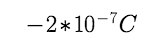is developed on polythene. What is the amount of mass, which is transferred to polythene.

Solution

From Q = ne, so the number of electron transffered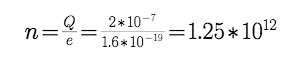Now mass of transffred electorn = n * mass of one electron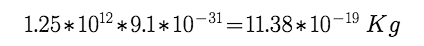Example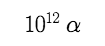– particles (Nuclei of helium) per second falls on a neutral sphere, calculate time in which sphere gets charged by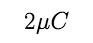Solution

Number of alpha – particles falling in t second =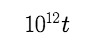Charge on alpha – particle = +2e , So charge incident in time t =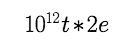given charge is  :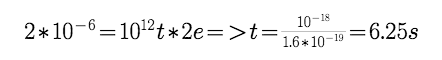t=\frac {{10}^{-18}} {1.6*{10}^{-19}}=6.25s"/>

Post By : Amit Rai 18 Apr, 2019 2682 views Physics